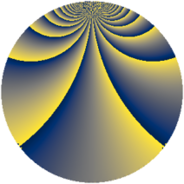# Properties

 Label 441.2.bgLevel $441$ Weight $2$ Character orbit 441.bg Rep. character $\chi_{441}(17,\cdot)$ Character field $\Q(\zeta_{42})$ Dimension $216$ Newform subspaces $1$ Sturm bound $112$ Trace bound $0$

# Related objects

## Defining parameters

 Level: $$N$$ $$=$$ $$441 = 3^{2} \cdot 7^{2}$$ Weight: $$k$$ $$=$$ $$2$$ Character orbit: $$[\chi]$$ $$=$$ 441.bg (of order $$42$$ and degree $$12$$) Character conductor: $$\operatorname{cond}(\chi)$$ $$=$$ $$147$$ Character field: $$\Q(\zeta_{42})$$ Newform subspaces: $$1$$ Sturm bound: $$112$$ Trace bound: $$0$$

## Dimensions

The following table gives the dimensions of various subspaces of $$M_{2}(441, [\chi])$$.

Total New Old
Modular forms 720 216 504
Cusp forms 624 216 408
Eisenstein series 96 0 96

## Trace form

 $$216 q - 16 q^{4} + 2 q^{7} + O(q^{10})$$ $$216 q - 16 q^{4} + 2 q^{7} + 12 q^{10} + 12 q^{16} - 6 q^{19} + 44 q^{22} + 26 q^{25} + 84 q^{28} - 6 q^{31} - 112 q^{34} + 60 q^{37} - 304 q^{40} + 20 q^{43} - 20 q^{46} - 86 q^{49} - 168 q^{52} - 84 q^{55} - 120 q^{58} - 2 q^{61} + 32 q^{64} + 22 q^{67} - 136 q^{70} - 6 q^{73} + 84 q^{76} + 2 q^{79} - 104 q^{82} + 96 q^{85} - 12 q^{88} + 58 q^{91} + 52 q^{94} + O(q^{100})$$

## Decomposition of $$S_{2}^{\mathrm{new}}(441, [\chi])$$ into newform subspaces

Label Dim. $$A$$ Field CM Traces $q$-expansion
$$a_2$$ $$a_3$$ $$a_5$$ $$a_7$$
441.2.bg.a $$216$$ $$3.521$$ None $$0$$ $$0$$ $$0$$ $$2$$

## Decomposition of $$S_{2}^{\mathrm{old}}(441, [\chi])$$ into lower level spaces

$$S_{2}^{\mathrm{old}}(441, [\chi]) \cong$$ $$S_{2}^{\mathrm{new}}(147, [\chi])$$$$^{\oplus 2}$$